Definitions of Square Dance Calls and Concepts

Index -->  Plus  |  A1  |  A2  |  C1  |  C2  |  C3A  |  C3B  |  C4  |  NOL  |
Definitions (Text Only) -->  Plus  |  A1  |  A2  |  C1  |  C2  |  C3A  |  C3B  |  C4  |  NOL  |
 Find call:

 \$B8@8l(B\$BJ?9T\$J(B Line \$B\$^\$?\$O(B, \$B0lHL2=\$5\$l\$?(B Thar \$B\$+\$i(B.

2. Centers Hinge;
3. Diamond Circulate;
4. Centers Hinge.

\$BJ?9T\$J(B Line \$B\$+\$i\$O(B \$BJ?9T\$J(B Line \$B\$G=*\$o\$j\$^\$9(B. Trade The Diamond \$B\$O(B 4 \$B%Q!<%H\$N%3!<%k\$G\$9(B.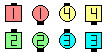Trade The Diamond\$B\$NA0(B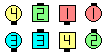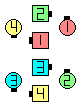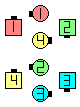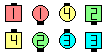Trade The Deucey(1/4)\$B\$N8e(B Centers Hinge(1/2)\$B\$N8e(B Diamond Circulate(3/4)\$B\$N8e(B Centers Hinge\$B\$N8e(B (\$B=*\$o\$j(B)

\$BCml9g(B, \$B%@%s%5!<\$O1&

Criss Cross The Diamond [C4]: \$BJ?9T\$J(B Wave \$B\$+\$i(B. Criss Cross The Deucey; Centers Hinge; Diamond Circulate; Centers Hinge. \$BJ?9T\$J(B Wave \$B\$G=*\$o\$j\$^\$9(B.Choreography for Trade The DiamondComments? Questions? Suggestions?